Aptitude Tests 4 Me

Non Verbal Logic

Detailed solution

47.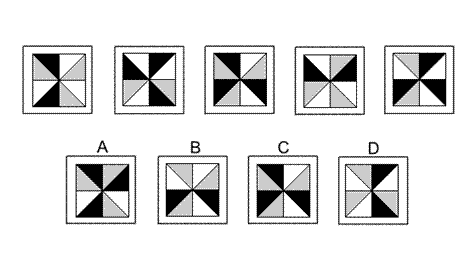Solution: A Explanation: In this question there are two rules that are applied simultaneously. The first rule is that the square of triangles is rotated by 90° anticlockwise each time. The second rule is that the colours of the triangles change as follows - black changes to grey, grey changes to white and white changes to black. When these two rules are applied simultaneously, the next diagram in the sequence is A.

48.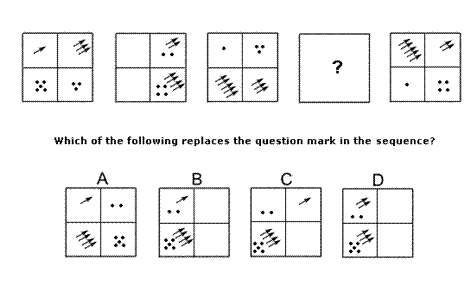Solution: B Explanation: In this question there are two groups of dots and two groups of arrows. The dots rotate one place anticlockwise around the grid each time and the number of dots follows the pattern 5 -->4 3 2 1 5etc. The arrows rotate one place clockwise around the grid each time and the number of arrows follows the pattern 1 -->2 3 4 5 1etc. When both these rules are applied simultaneously to both sets of dots and arrows, the correct answer for the missing diagram of the sequence must be B.

49.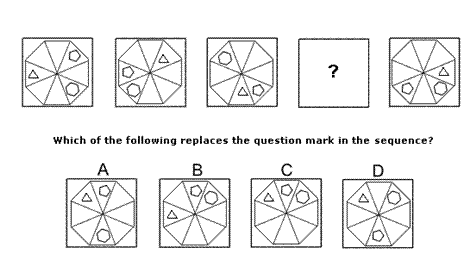Solution: C Explanation: In this question there is a triangle, a pentagon and a hexagon that rotate around the eight triangles that make up a regular octagon, each according to its own rule. The triangle always rotates by 3 places clockwise. When this rule is applied, the correct answer for the missing diagram of the sequence could be A, C or D. The pentagon always rotates five places clockwise. When this rule is also applied, the correct answer for the missing diagram of the sequence could be A or C. The hexagon always rotates six places anticlockwise. When this rule is also applied, the correct answer for the missing diagram of the sequence must be C.

50.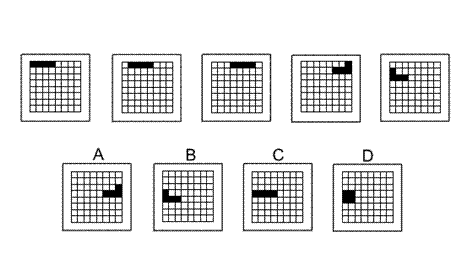Solution: B Explanation: In this question there is a 'train' of four black squares that zigzags its way down the rows of the grid. The first time, the train' moves forwards 1 place, the second time 2 (= 21) places, the third time 4 (= 22) places, etc. The number of places it moves forwards doubles each time. For the next diagram of the sequence, therefore, it should move forward 16 (= 24) places, and the correct answer is B.

51.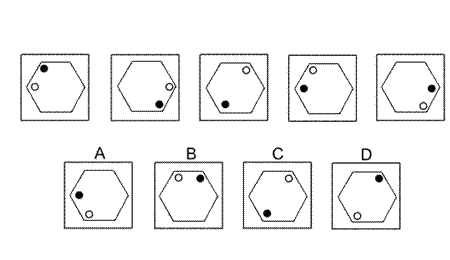1 2 3 4 5 6 7 8 9 10 11 12 13 14 15 16 17 18 19 20 21 22 23 24 25 26 27 28 29 30 31 32 33 34 35 36 37 38 39 40 41 42 43 44 45 46 47 48 49 50 51 52 53 54 55 56 57 58 59 60 61 62 63 64 65 66 67 68 69 70 71 72 73 74 75 76 77 78 79 80 81 82 83 84 85 86 87 88Passage ReadingVerbal LogicNon Verbal LogicNumerical LogicData InterpretationReasoningAnalytical AbilityBasic NumeracyAbout UsContactPrivacy PolicyMajor TestsFAQ